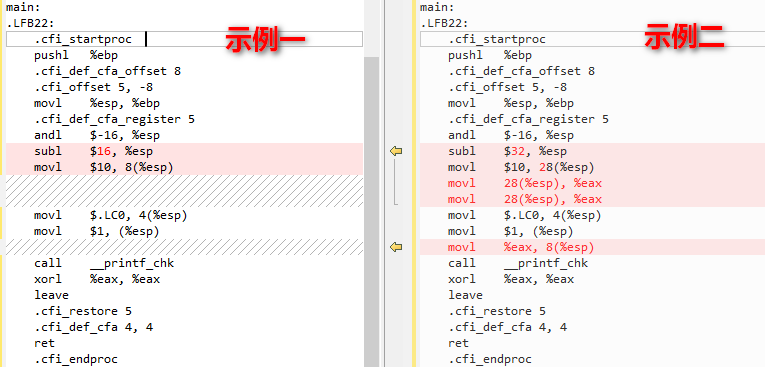# C语言再学习 -- 关键字volatile

Indicates that a variable can be changed by a background routine.

Keyword volatile is an extreme opposite of const.It indicates that a variable may be changed in a way which is absolutely unpredictable by analysing the normal program flow (for example, a variable which may be changed by an interrupt handler). This keyword uses the following syntax:

volatile data-definition;

Every reference to the variable will reload the contents from memory rather than take advantage of situations where a copy can be in a register.

volatile data-definition;

volatile应用比较多的场合，在中断服务程序和cpu相关寄存器的定义

volatile 用于相关寄存器定义

//编译led.c文件

#define GPC1CON *((volatile unsigned int*)0xE0200080)
#define GPC1DAT *((volatile unsigned int*)0xE0200084)
#define GPC1PUD *((volatile unsigned int*)0xE0200088)
//隐式声明
void delay (unsigned int);
void led_test (void) {
//配置相应管脚为输出功能 GPC1_3
GPC1CON &= ~(0x0f << 12);
GPC1CON |= (1 << 12);
//GPC1_4为输出功能
GPC1CON |= (1 << 16);
//禁止内部上拉下拉功能
GPC1PUD &= ~(0x03 << 6);
GPC1PUD &= ~(0x03 << 8);
while (1) {
//灯亮
GPC1DAT |= (1 << 3);
GPC1DAT |= (1 << 4);
delay (0x100000);
//灯灭
GPC1DAT &= ~(1 << 3);
GPC1DAT &= ~(1 << 4);
delay (0x100000);
}
}

void delay (unsigned int n) {
unsigned int i = 0;
for (i = n; i != 0; i--);
}

arm-linux-gcc -c led.c -o led.o –nostdlib

//示例一
#include <stdio.h>
int main (void)
{
int i = 10;
int a = i; //优化
int b = i;

printf ("i = %d\n", b);
return 0;
}

//编译优化、查看汇编
gcc -O2 -S test.c
cat test.s

.file	"test.c"
.section	.rodata.str1.1,"aMS",@progbits,1
.LC0:
.string	"i = %d\n"
.section	.text.startup,"ax",@progbits
.p2align 4,,15
.globl	main
.type	main, @function
main:
.LFB22:
.cfi_startproc
pushl	%ebp
.cfi_def_cfa_offset 8
.cfi_offset 5, -8
movl	%esp, %ebp
.cfi_def_cfa_register 5
andl	$-16, %esp subl$16, %esp
movl	$10, 8(%esp) movl$.LC0, 4(%esp)
movl	1, (%esp) call __printf_chk xorl %eax, %eax leave .cfi_restore 5 .cfi_def_cfa 4, 4 ret .cfi_endproc .LFE22: .size main, .-main .ident "GCC: (Ubuntu/Linaro 4.6.3-1ubuntu5) 4.6.3" .section .note.GNU-stack,"",@progbits  //示例二 #include <stdio.h> int main (void) { volatile int i = 10; int a = i; //未优化 int b = i; printf ("i = %d\n", b); return 0; }  //编译优化、查看汇编 gcc -O2 -S test.c cat test.s .file "test.c" .section .rodata.str1.1,"aMS",@progbits,1 .LC0: .string "i = %d\n" .section .text.startup,"ax",@progbits .p2align 4,,15 .globl main .type main, @function main: .LFB22: .cfi_startproc pushl %ebp .cfi_def_cfa_offset 8 .cfi_offset 5, -8 movl %esp, %ebp .cfi_def_cfa_register 5 andl-16, %esp
subl	$32, %esp movl$10, 28(%esp)
movl	28(%esp), %eax
movl	28(%esp), %eax
movl	$.LC0, 4(%esp) movl$1, (%esp)
movl	%eax, 8(%esp)
call	__printf_chk
xorl	%eax, %eax
leave
.cfi_restore 5
.cfi_def_cfa 4, 4
ret
.cfi_endproc
.LFE22:
.size	main, .-main
.ident	"GCC: (Ubuntu/Linaro 4.6.3-1ubuntu5) 4.6.3"
.section	.note.GNU-stack,"",@progbitsvolatile 指出 i 是随时可能发生变化的，每次使用它的时候必须从 i的地址中读取，因而编译器生成的汇编代码会重新从i的地址读取数据放在 b 中。而优化做法是，由于编译器发现两次从 i读数据的代码之间的代码没有对 i 进行过操作，它会自动把上次读的数据放在 b 中。而不是重新从 i 里面读。这样以来，如果 i是一个寄存器变量或者表示一个端口数据就容易出错，所以说 volatile 可以保证对特殊地址的稳定访问

#include <stdio.h>
#include <sys/timeb.h>
long long getSystemTime() {
struct timeb t;
ftime(&t);
return 1000 * t.time + t.millitm;
}

#define TIME 1000000000

int main(void)
{

volatile int a, b = TIME; /* volatile修饰变量 */
int x, y = TIME;          /* 一般变量   */
long long start = 0, end = 0;

start=getSystemTime();
for (a = 0; a < b; a++);
end=getSystemTime();
printf("vloatile修饰变量用时: %lld ms\n", end - start);

start=getSystemTime();
for (x = 0; x < y; x++);
end=getSystemTime();
printf("一般变量用时: %lld ms\n", end - start);

return 0;
}  
编译：gcc test.c

vloatile修饰变量用时: 3738 ms

优化编译：gcc -O2 test.c

vloatile修饰变量用时: 3550 ms

for(int i=0; i<100000; i++);

for(volatile int i=0; i<100000; i++);

1、编译器优化介绍：

，为提高机器整体性能，在硬件上引入硬件高速缓存Cache，加速对内存的访问。另外在现代CPU中指令的执行并不一定严格按照顺序执行，没有相关性的指令可以乱序执行，以充分利用CPU的指令流水线，提高执行速度。以上是硬件级别的优化。再看软件一级的优化：一种是在编写代码时由程序员优化，另一种是由编译器进行优化。编译器优化常用的方法有：将内存变量缓存到寄存器；调整指令顺序充分利用CPU指令流水线，常见的是重新排序读写指令。对常规内存进行优化的时候，这些优化是透明的，而且效率很好。由编译器优化或者硬件重新排序引起的问题的解决办法是在从硬件（或者其他处理器）的角度看必须以特定顺序执行的操作之间设置内存屏障（memory barrier），Linux 提供了一个宏解决编译器的执行顺序问题。
void Barrier(void)

2、volatile总是与优化有关，编译器有一种技术叫做数据流分析，分析程序中的变量在哪里赋值、在哪里使用、在哪里失效，分析结果可以用于常量合并，常量传播等优化，进一步可以消除一些代码。但有时这些优化不是程序所需要的，这时可以用volatile关键字禁止做这些优化。

volatile的本意是“易变的” 因为访问寄存器要比访问内存单元快的多,所以编译器一般都会作减少存取内存的优化，但有可能会读脏数据。当要求使用volatile声明变量值的时候，系统总是重新从它所在的内存读取数据，即使它前面的指令刚刚从该处读取过数据。精确地说就是，遇到这个关键字声明的变量，编译器对访问该变量的代码就不再进行优化，从而可以提供对特殊地址的稳定访问；如果不使用valatile，则编译器将对所声明的语句进行优化。（简洁的说就是：volatile关键词影响编译器编译的结果，用volatile声明的变量表示该变量随时可能发生变化，与该变量有关的运算，不要进行编译优化，以免出错

1、并行设备的硬件寄存器（如：状态寄存器）

int  *output = (unsigned  int *)0xff800000;//定义一个IO端口；
int   init(void)
{
int i;
for(i=0;i< 10;i++){
*output = i;
}
}

int  init(void)
{
*output = 9;
}

//编译led.c文件

#define GPC1CON *((volatile unsigned int*)0xE0200080)
#define GPC1DAT *((volatile unsigned int*)0xE0200084)
#define GPC1PUD *((volatile unsigned int*)0xE0200088)
//隐式声明
void delay (unsigned int);
void led_test (void) {
//配置相应管脚为输出功能 GPC1_3
GPC1CON &= ~(0x0f << 12);
GPC1CON |= (1 << 12);
//GPC1_4为输出功能
GPC1CON |= (1 << 16);
//禁止内部上拉下拉功能
GPC1PUD &= ~(0x03 << 6);
GPC1PUD &= ~(0x03 << 8);
while (1) {
//灯亮
GPC1DAT |= (1 << 3);
GPC1DAT |= (1 << 4);
delay (0x100000);
//灯灭
GPC1DAT &= ~(1 << 3);
GPC1DAT &= ~(1 << 4);
delay (0x100000);
}
}

void delay (unsigned int n) {
unsigned int i = 0;
for (i = n; i != 0; i--);
}

arm-linux-gcc -c led.c -o led.o –nostdlib

#define GPC1CON *((volatile unsigned int*)0xE0200080)   怎么理解？

GPC1CON 为寄存器名称、0xE0200080 为寄存器地址、(volatile unsigned int*) 为强制类型转换。

2、一个中断服务子程序中会访问到的非自动变量（Non-automatic variables)

static int i=0; //i 为非自动变量
int main(void)
{
...
while (1){
if (i) dosomething();
}
｝
/* Interrupt service routine. */
void ISR_2(void)
{
i=1;
}

3、多线程应用中被几个任务共享的变量

volatile  BOOL  bStop  =  FALSE;  //bStop  为共享全局变量
(1) 在一个线程中：
while(  !bStop  )  {  ...  }
bStop  =  FALSE;
return;

(2) 在另外一个线程中，要终止上面的线程循环：
bStop  =  TRUE;
while(  bStop  ); 

volatile 常见的几个面试题

1、一个参数既可以是const还可以是volatile吗？

2、一个指针可以是 volatile 吗？

3、下面的函数有什么错误：

int square(volatile int *ptr)
{
return *ptr * *ptr;
} 

  int square(volatile int *ptr)
{
int a,b;
a = *ptr;
b = *ptr;
return a * b;
} 
由于*ptr的值可能被意想不到地该变，因此a和b可能是不同的。结果，这段代码可能返不是你所期望的平方值！正确的代码如下：

long square(volatile int *ptr)
{
int a;
a = *ptr;
return a * a;
}

volatile 关键字是一种类型修饰符，用它声明的类型变量表示可以被某些编译器未知的因素更改。volatile 提醒编译器它后面所定义的变量随时都有可能改变，因此编译后的程序每次需要存储或读取这个变量的时候，都会直接从变量地址中读取数据。如 果没有 volatile 关键字，则编译器可能优化读取和存储，可能暂时使用寄存器中的值，如果这个变量由别的程序更新了的话，将出现不一致的现象。所以遇到这个关键字声明的变量，编译器对访问该变量的代码就不再进行优化，从而可以提供对特殊地址的稳定访问

©️2019 CSDN 皮肤主题: 程序猿惹谁了 设计师: 上身试试